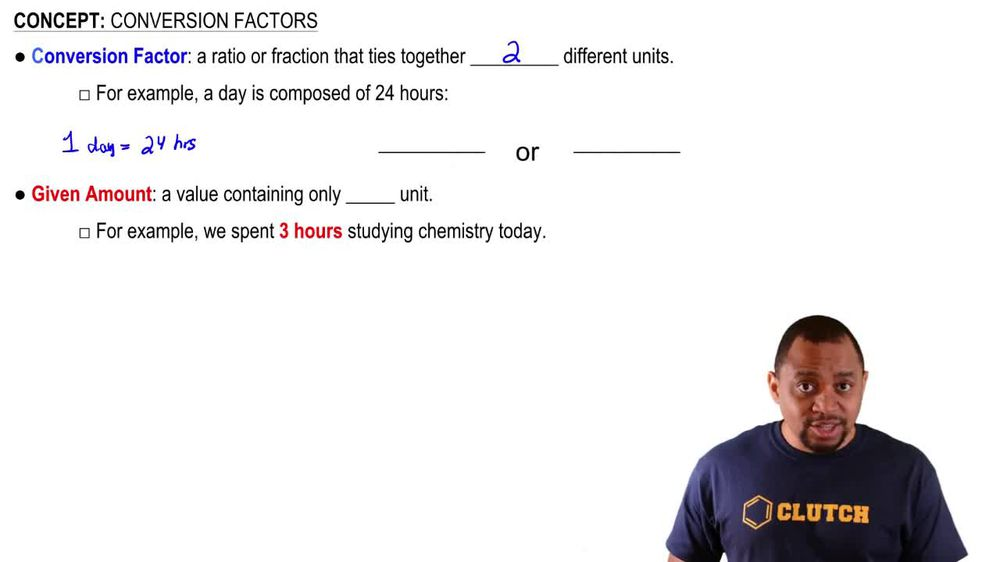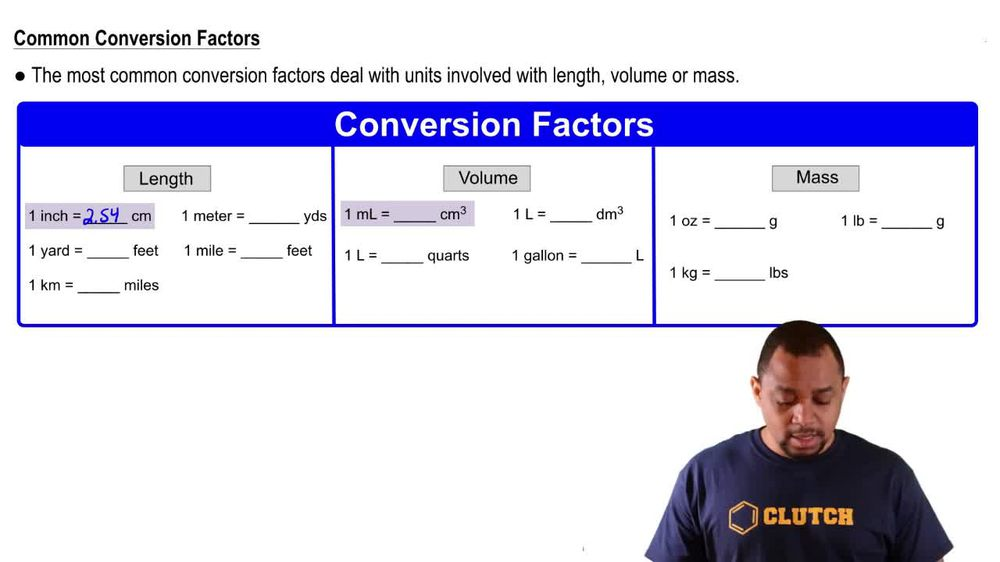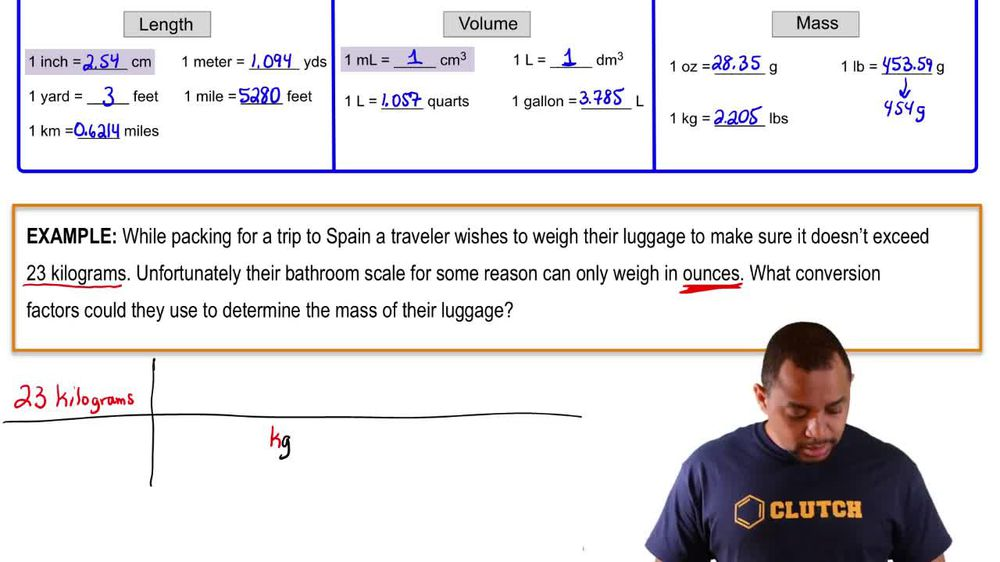Start typing, then use the up and down arrows to select an option from the list.
1. 1. Intro to General Chemistry2. Conversion Factors
Problem

# Which is larger in each pair, and by approximately how much? (a) A liter or a quart

Relevant Solution45s
Play a video:
Hey, everyone were asked is a fluid ounce larger than a pint. If so, by how much to answer this question, we need to recall the conversion factors we've learned And this is going to be that one pint is equivalent to 16 fluid ounces. Looking at this conversion, we can already tell that a pint is larger than a fluid ounce, So our answer here is going to be no, a pint is about 16 times larger, then a fluid ounce, and this is going to be our final answer. Now, I hope that made sense and let us know if you have any questions.